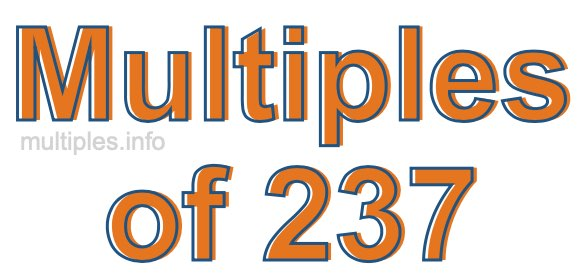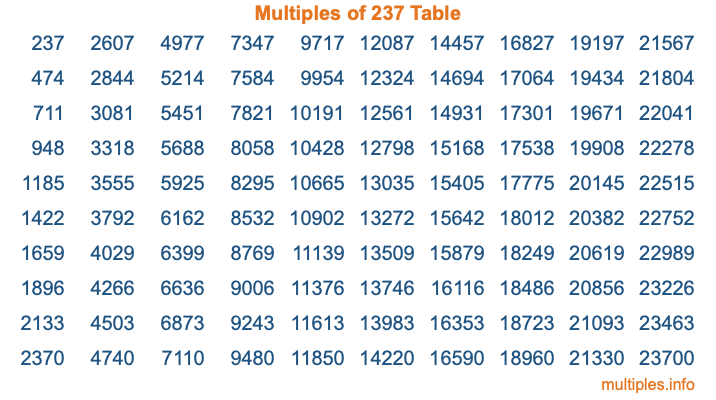Multiples of 237Welcome to the Multiples of 237 page. Here we will first teach you everything you will ever need to know about the multiples of 237, and then give you a study guide summary of everything we taught you to make sure you remember it all. Use this page to look up facts and learn information about the multiples of 237. This page will make you a multiples of two hundred thirty-seven expert!

Definition of Multiples of 237
Multiples of 237 are all the numbers that when divided by 237 equal an integer. Each of the multiples of 237 are called a multiple. A multiple of 237 is created by multiplying 237 by an integer.

Therefore, to create a list of multiples of 237, you start with 1 multiplied by 237, then 2 multiplied by 237, then 3 multiplied by 237, and so on for as long as you want. Thus, the list of the first five multiples of 237 is 237, 474, 711, 948, and 1185. To see a larger list of multiples of 237, see the printable image of Multiples of 237 further down on this page. We also have a category where you can choose any nth multiple of 237.

Multiples of 237 Checker
The Multiples of 237 Checker below checks to see if any number of your choice is a multiple of 237. In other words, it checks to see if there is any number (integer) that when multiplied by 237 will equal your number. To do that, we divide your number by 237. If the the quotient is an integer, then your number is a multiple of 237.

Is  a multiple of 237?

Least Common Multiple of 237 and ...
A Least Common Multiple (LCM) is the lowest multiple that two or more numbers have in common. This is also called the smallest common multiple or lowest common multiple and is useful to know when you are adding our subtracting fractions. Enter one or more numbers below (237 is already entered) to find the LCM.

Check out our LCM Calculator if you need more details about the Least Common Multiple or if you need the LCM for different numbers for adding and subtraction fractions.

nth Multiple of 237
As we stated above, 237 is the first multiple of 237, 474 is the second multiple of 237, 711 is the third multiple of 237, and so on. Enter a number below to find the nth multiple of 237.

th multiple of 237

Multiples of 237 vs Factors of 237
237 is a multiple of 237 and a factor of 237, but that is where the similarities end. All postive multiples of 237 are 237 or greater than 237. All positive factors of 237 are 237 or less than 237.

Below is the beginning list of multiples of 237 and the factors of 237 so you can compare:

Multiples of 237: 237, 474, 711, 948, 1185, etc.

Factors of 237: 1, 3, 79, 237

As you can see, the multiples of 237 are all the numbers that you can divide by 237 to get a whole number. The factors of 237, on the other hand, are all the whole numbers that you can multiply by another whole number to get 237.

It's also interesting to note that if a number (x) is a factor of 237, then 237 will also be a multiple of that number (x).

Multiples of 237 vs Divisors of 237
The divisors of 237 are all the integers that 237 can be divided by evenly. Below is a list of the divisors of 237.

Divisors of 237: 1, 3, 79, 237

The interesting thing to note here is that if you take any multiple of 237 and divide it by a divisor of 237, you will see that the quotient is an integer.

Multiples of 237 Table
Below is an image of the first 100 multiples of 237 in a table. The table is in chronological order, column by column. The first column has the first ten multiples of 237, the second column has the next ten multiples of 237, and so on.The Multiples of 237 Table is also referred to as the 237 Times Table or Times Table of 237. You are welcome to print out our table for your studies.

Negative Multiples of 237
Although not often discussed or needed in math, it is worth mentioning that you can make a list of negative multiples of 237 by multiplying 237 by -1, then by -2, then by -3, and so on, to get the following list of negative multiples of 237:

-237, -474, -711, -948, -1185, etc.

Multiples of 237 Summary
Below is a summary of important Multiples of 237 facts that we have discussed on this page. To retain the knowledge on this page, we recommend that you read through the summary and explain to yourself or a study partner why they hold true.

There are an infinite number of multiples of 237.

A multiple of 237 divided by 237 will equal a whole number.

237 divided by a factor of 237 equals a divisor of 237.

The nth multiple of 237 is n times 237.

The largest factor of 237 is equal to the first positive multiple of 237.

237 is a multiple of every factor of 237.

237 is a multiple of 237.

A multiple of 237 divided by a divisor of 237 equals an integer.

237 divided by a divisor of 237 equals a factor of 237.

Any integer times 237 will equal a multiple of 237.

Multiples of a Number
Here you can get the multiples of another number, all with the same attention to detail as we did for multiples of 237 on this page.

Multiples of
Multiples of 238
Did you find our page about multiples of two hundred thirty-seven educational? Do you want more knowledge? Check out the multiples of the next number on our list!